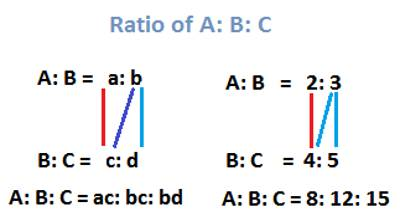A ratio is a statement of how two numbers compare. It is a comparison of the size of one number to the size of another number. It tells how one number is related to another number.

A ratio may be written as A:B or A/B or by the phrase “A to B”

A ratio of 1:5 says that the second number is five times as large as the first.

The following steps will allow determination of a number when one number and the ratio between the numbers is given.

Example: Determine the value of B if A=6 and the ratio of A:B = 2:5

• Determine how many times the number A is divisible by the corresponding portion of the ratio. (6/2 = 3)
• Multiply this number by the portion of the ratio representing B (3*5 = 15)
• Therefore if the ratio of A:B is 2:5 and A = 6 then B = 15.

How to Determine a Ratio

Ratios represent how one quantity is related to another quantity. A ratio may be written as A:B or A/B or by the phrase “A to B”. A ratio of 1:5 says that the second quantity is five times as large as the first.

The following steps will allow a ratio to be the determination if two numbers are known.

Example: Determine the ratio of 24 to 40.

• Divide both terms of the ratio by the greatest common factor (24/8 = 3, 40/8=5)
• State the ratio. (The ratio of 24 to 40 is 3:5).Expressing a Ratio

Mathematicians and scientists use one of three conventions to express a ratio. Suppose you have two numbers A and B. You can express the ratio between them as:

A:B

A to B

A/B

When reading the ratio aloud, you always say “A to B.” The term for A is the antecedent, and the term for B is the consequent.

As an example, consider a grade school class which has 32 students, 17 of whom are girls and 15 of which are boys. The ratio of girls to boys can be written as 17:15, 17 to 15 or 17/15, while the ratio of boys to girls is 15:17, 15 to 17 or 15/17. The classroom has 32 students, so the ratio of girls to the total number of students is 17:32, and the ratio of boys to the total number of students is 15:32.

When comparing part of a whole to the whole, you can convert the ratio to a percentage by expressing it in fractional form, dividing the antecedent by the consequent and multiplying by 100. In our example, we find that the class is 17/32 x 100 = 53% female and 15/32 x 100 = 47% male. In terms of percentages, the ratio of girls to boys is 53:47, and the ratio of boys to girls is 47:53.

Information Source: